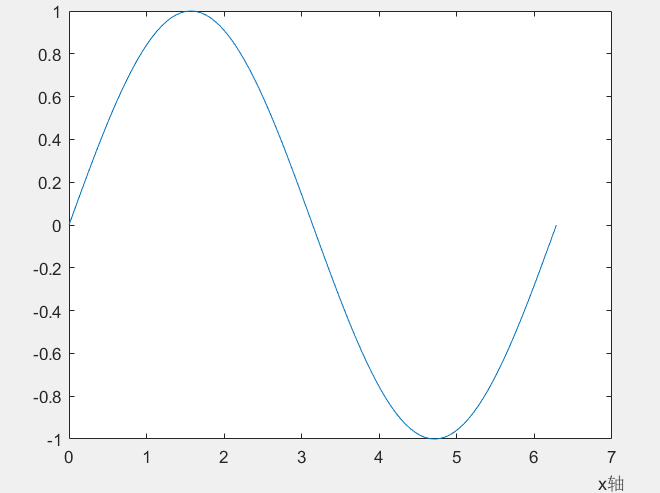• ## matlabxlabel位置设置

千次阅读 2020-03-20 17:54:40
xlabel中也有position用法 xlabel(‘time’,‘position’,[900,1870],‘FontSize’,12); 此时‘time’在你设置的位置 还有一种用法是类似图像的用法 pos=axis;%取得当前坐标轴的范围，即[xmin xmax ymin ymax] ...
xlabel(‘time’,‘FontSize’,12);
如果没有设置位置，默认是在中间
在xlabel中也有position用法
xlabel(‘time’,‘position’,[900,1870],‘FontSize’,12);
此时‘time’在你设置的位置
还有一种用法是类似图像的用法
pos=axis;%取得当前坐标轴的范围，即[xmin xmax ymin ymax]
xlabel(‘time’,‘FontSize’,12, ‘Position’,[pos(2) pos(3)])
x=0:pi/50:2*pi;
y=sin(x);
plot(x,y);
pos=axis;%取得当前坐标轴的范围，即[xmin xmax ymin ymax]
xlabel('x轴','position',[pos(2) 1.15*pos(3)]);%设置x轴标签的文本在图的右下方，1.15这个值根据自己的需要可以调整
形成的图参考链接2：http://blog.sina.com.cn/s/blog_7e6270010101nt0r.html#commonComment


展开全文• 原文地址：http://blog.sina.com.cn/s/blog_8fc890a20102v0sl.html 适用于title、xlabel、ylabel、text等命令。 首先附上 Tex 字符即函数表 注：没表示出来的说明太复杂，一般不常...


原文地址：http://blog.sina.com.cn/s/blog_8fc890a20102v0sl.html

适用于title、xlabel、ylabel、text等命令。

首先附上 Tex 字符即函数表
注：没表示出来的说明太复杂，一般不常用

函数字符

代表符号

函数字符

代表符号

函数字符

代表符号

\alpha

α

\upsilon

Υ

\sim

~

\beta

β

\phi

Φ

\leq

≤

\gamma

γ

\chi

Χ

\infty

∞

\delta

δ

\psi

Ψ

\clubsuit

一个梅花

\epsilon

ϵ

\omega

Ω

\diamondsuit

\zeta

ζ

\Gamma

Γ

\heartsuit

恒等号

\eta

η

\Delta

Δ

黑桃

\theta

θ

\Theta

Θ

\leftrightarrow

↔

\vartheta

ϑ

\Lambda

Λ

\leftarrow

←

\iota

ι

\Xi

Ξ

\uparrow

↑

\kappa

κ

\Pi

Π

\rightarrow

→

\lambda

λ

\Sigma

Σ

\downarrow

↓

\mu

μ

\Upsilon

Υ

\circ

°

\nu

ν

\Phi

Φ

\pm

±

\xi

ξ

\Psi

Ψ

\geq

≥

\pi

π

\Omega

Ω

\propto

∝

\rho

ρ

\forall

∀

\partial

∂

\sigma

σ

\exists

∃

\bullet

∙

\varsigma

ς

\ni

\div

÷

\tau

τ

\cong

≅

\neq

≠

\equiv

≡

\approx

≈

\aleph

ℵ

\Im

\Re

\wp

\otimes

⊗

\oplus

⊕

\oslash

\cap

∩

\cup

∪

\supseteq

⊇

\supset

⊂

\subseteq

⊆

\subset

⊃

\int

∫

\in

∈

\o

ο

\rfloor

\lceil

\nabla

\lfloor

\cdot

\ldots

\perp

\neg

\prime

\wedge

\times

\0

∅

\rceil

\surd

\mid

|

\vee

\varpi

\langle

\rangle

Tex 字符字体的设置

\bf  是 粗体                      \it  是斜体                         \sl 是斜体字，少用
\rm 是正常字体                \fontname{宋体}              \fontsize{16}
\color{red(green yellow magenta blue black white cyan gray barkGreen orange lightblue)}
_  和 ^ 分别是下标和上标

MATLAB 与 LaTex

格式一般通用，即

text('Interpreter','latex','String','$数学公式$', 'Position',[0.5 0.6],'FontSize',24)
xlabel('$数学公式$','Interpreter','LaTex');

h = legend('sin(x)__','2*sin(x)__');
h1 = findobj(get(h,'Children'),'String','sin(x)__');
set(h1,'String','$sin(\hat{x})$','Interpreter','LaTex');

title('$How \ to \ use \ latex \ in \ figure$','Interpreter','LaTex');

其中，String后面用了   '$数学公式$'，其实一共有三种：

a.    '\( 数学公式 )\'
b.    '$数学公式$'
c.    '$$数学公式$$'

第一种不知为什么实验不成功呢。。
第二种和第三种都可以用，但是是有区别的，各位可以将下面的积分，求极限的符号带入，分别用第二种和第三种来作图，会发现不一样，个人倾向于使用第三种。

数学公式的常用命令有：
1. 如果是Tex字符，直接打出 \alpha 即可。
2. 求和          \sum_{i=1}^{n} x_{i}                         % n 和 i 也可以不用大括号
3. 积分          \int_0^1                                            % 0 到 1 上的积分
4. 求极限      limn→∞limn→∞       % 无穷符号在正下方
$\lim_{n \rightarrow \infty}$           % 无穷符号在右下角
5. 分式         \frac{1}x                                            %
1/x
6. 根式         \sqrt{x}                                              % 根号x
7. 上划线      \overline{x}                                      % x上面有一横
8. 下划线      \underline{x}
9. 卧式花括号    \overbrace{x+y+z+w}
10. 仰式花括号 a+\underbrace{b+c+d}
11. 戴帽命令   \hat{o}\
\ \check{o}\ \ \breve{o}
\widehat{A+B} \ \ \widetilde{a+b}
\vec{\imath}+\vec{\jmath}=\vec{k}
12. 堆砌命令:   y\stackrel{\rm
def}{=} f(x) \stackrel{x\rightarrow 0}{\rightarrow} A   %有用
13.  省略号:      \cdots
\ldots \vdots \ddots


展开全文• 1. plot(x,y); %x y 为相应点集 2.plot(x,y1,x,y2); % 在一个窗口下绘制多条曲线之方法一 3.hold on %在一个窗口下绘制多条曲线之方法二 ... xlabel('x_axis_name'); %设置x y轴名称  ylabel('x_axis
1. plot(x,y); %x y 为相应点集
2.plot(x,y1,x,y2); % 在一个窗口下绘制多条曲线之方法一
3.hold on  %在一个窗口下绘制多条曲线之方法二
plot(x,y1);
plot(x,y2);
hold off
4.plot后
xlabel('x_axis_name'); %设置x y轴名称
ylabel('x_axis_name');
title('name'); %设置图名称
5.线型和颜色
线型（线方式）： - 实线 :点线 -. 虚点线 - - 波折线
线型（点方式）：. 圆点 +加号 * 星号 x x形 o 小圆
线条粗细：plot(x,y,'r','linewidth',4);
颜色： r红； g绿； b蓝； c青  m紫； k黑； w白；y黄；
例子：
plot(x,y1,’b:+’,x,y2,’g-.*’);
6.加图例legend
legend(字符串1，字符串2，字符串3，…，参数);  %其中字符串为画图顺序依次标注，参数说明如下：
参数字符串                              含  义
0                             尽量不与数据冲突，自动放置在最佳位置
1                              放置在图形的右上角
2                              左上角
3                             左下角
4                             右下角
-1                            图形窗外
7.设置背景色
set(gcf,'color','none'); %无背景
set(gcf,'color',[0,0,0]); %背景色为黑
set(gcf,'color',[1,1,1]); %背景色为白

展开全文• hold on plot xlabel(); ylabel(); legend(); title(); print
• surf函数用法： 例如1：绘制山峰曲面 close all >> [X,Y,Z]=peaks(30); >> surf(X,Y,Z); >> title('山峰表面'); >> xlabel('x-axis'); >> ylabel('y-axis'); >> zlabel('z-...
• 二维高层绘图的基本函数 plot函数 ...特殊用法： 标注： 基本标注函数：  title  xlabel  ylabel  text  legend 特殊字符：  LaTeX 二维绘图的辅助操作 标注：图形名称
• 基本东西 legend图例，ylabel y轴名称，xlabel x轴名称 gpname 不同组的名字等等。 一般set函数就行。set(句柄，属性名1，属性值1，属性名2，属性值2，…) eg：set(gca,'XTickLabel',{'1','2'); 1. 折线图，使用...
• 可以用matlab提供的坐标轴设置函数axis进行设置（对当前坐标轴进行设置），具体用法如下： plot(x, y); // 画图后用axis函数设置坐标轴的范围 axis([xmin xmax ymin ymax]); % 设置坐标轴在指...
• xlabel(‘string’) ylabel(‘string’) 2.图名 title(‘string’) 3.图例 legend(‘string1’,‘string’) 4.文字注释 text(x,y,‘string’) 图形控制命令 1.坐标控制 axis([xmin,xmax,ymin,ymax]) %x的取值，y的...
• 关于MATLAB 直方图 整理记录histogram2 —三维直方图的一些用法及拓展应用 散点绘制直方图 h = histogram2(Xe,Ye,10,'FaceColor','flat'),title('Entries'),xlabel('Xe'),ylabel('Ye'),colorbar,grid on Xe Ye，...cloudera
• MATLAB：绘图坐标轴的设置1. 坐标轴基本操作1.1 基本格式1.2 常见用法2. 刻度基本操作3. 两侧坐标轴 1. 坐标轴基本操作 1.1 基本格式 %某轴的名称设置 xlabel(‘x轴’,‘FontName’,‘宋体’,‘FontSize’,8,...
• 一、用法 1、三维曲线绘制函数为 plot3(x,y,z): 表示绘制一条三维曲线,其中x,y,z为三个相同维数的向量,函数绘出这些向量的所表示点的曲线 2、plot3(x1,y1,z1,c1,x2,y2,z2,c2)： 表示按照坐标对向量绘制多条曲线，...
• MATLAB绘图 二绘制图形的辅助操作给图形添加标注坐标控制图形保持图形窗口的分割 绘制图形的辅助操作 给图形添加标注 title(图形标题) xlabel(x轴说明) ylabel(y轴说明) text(x, y, 说明) legend(图例1, 图例2, …)...
• ## matlab学习（三）三维曲线和曲面

万次阅读 多人点赞 2019-04-06 15:10:38
plot3函数的基本用法 plot3(x, y, z) 其中，参数x、y、z组成一组曲线的坐标。 样例一：绘制一条空间折线。 x=[0.2, 1.5, 2.5]; y=[1.3, 2.8, 1.1]; z=[0.4, 1.2, 1.6]; plot3(x, y, z) xlabel('Variable X'); ...
• 在使用Matlab绘图的过程中，会经常使用到绘图的功能，今天总结一下大概的用法，以及一个之前没有遇到问题的解决方法。 1.常用的绘图功能 x = (1:50); y = sin(x); plot(x,y,'-r*'); xlabel('x name','fontsize',...
• 子图（类似MATLAB） ax = fig.add_subplot(221) 设置图参数 ax.set(xlim=[0.5, 4.5], ylim=[-2, 8], title='An Example Axes', ylabel='Y-Axis', xlabel='X-Axis') 多子图一次性设置 fig, axes = plt....
• clc,clear,close all; %清除命令，清空工作区，关闭...xlabel("X轴"); %设置X轴标签 ylabel("Y轴"); %设置Y轴标签 print(h,'-djpeg','1.jpg'); %保存成本地文件 printf用法之print(图形句柄,存储格式,文件名); 图形...
• 想试下MKL FFT的用法以及参数设置，选了两组数据和MATLAB运行测试比对。 实数范围内的FFT clear all close all Fs = 1000; T = 1/Fs; L = 1024; t = (0:L-1)*T; x = 0.5*sin(2*pi*15*t)+2*sin(2*pi*40*t); ...
• Matlab绘制三维图mesh函数：绘制网格图例1例2mashc函数mashz函数 mesh函数：绘制网格图 mesh(x, y, z)的用法，x是n维向量，y是m维向量，z是m*n的矩阵 X = [1,2,4] Y = [3,5] Z = [4,8,10;5,9,13] mesh(X,Y,Z) % (X(j...
• matlab画图显示XY轴名称xlabel(‘x轴的名称’);ylabel(‘y轴的名称’);matlab中plot()画图时如何指定X Y轴的范围可以用matlab提供的坐标轴设置函数axis进行设置(对当前坐标轴进行设置)，具体用法如下：plot(x, y); /...# matlabxlabel用法matlab 订阅